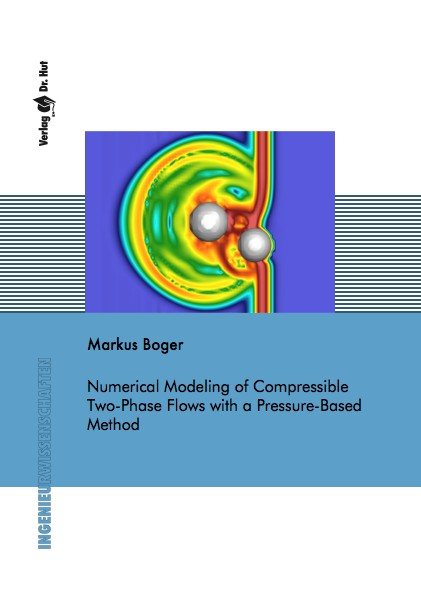Datenbestand vom 10. Dezember 2019aktualisiert am 10. Dezember 2019

# ISBN 978-3-8439-1514-4Euro 84,00 inkl. 7% MwSt

Markus Boger
Numerical Modeling of Compressible Two-Phase Flows with a Pressure-Based Method

193 Seiten, Dissertation Universität Stuttgart (2014), Softcover, A5

## Zusammenfassung / Abstract

This work is directed towards the direct numerical simulation (DNS) of compressible and incompressible multiphase flows. The main objective is the extension of an incompressible two-phase solver to the compressible flow regime.

The starting point is the existing multiphase solver FS3D for the simulation of three-dimensional, incompressible flows. For this code, the topic of surface tension modeling is looked at in detail with respect to the so-called parasitic currents. A balanced-force algorithm is presented that considerably reduces the unphysical parasitic currents by several orders of magnitude.

The main focus of this thesis is on the extension of an incompressible, pressure-based numerical approach to the simulation of compressible multiphase flows. For this purpose, the physical and mathematical background of the governing equations for incompressible and compressible flows is discussed and the transition from the compressible to the incompressible regime is addressed. In this context, fundamental investigations for the coupling of compressible and incompressible flow regions are presented in one space dimension and several iterative coupling schemes are derived and validated with the help of generic test cases.

The pressure-based Multiple Pressure Variables (MPV) method that allows the extension of an incompressible flow solver to the compressible regime is presented. The numerical scheme builds upon an asymptotic pressure decomposition taking into account the different roles of pressure for incompressible and compressible flows.

At first, the MPV method is introduced for single-phase flows and its extension to compressible two-phase flows is presented. In this context, the treatment of the material interface between the two fluids and the corresponding jump in the material properties and the equations of state play a crucial role. While the present approach numerically smears the density jump between the two phases, the thermodynamic transition is a sharp one. Contrary to many density-based two-phase flow solvers, the MPV scheme does not suffer from oscillations in pressure and velocity at the interface location due to the use of pressure as primary variable. A detailed analysis of this behavior is presented. Finally, the MPV scheme proves to accurately solve one-dimensional single- and two-phase Riemann problems as well as three-dimensional shock-droplet interactions.